# Test: Performance of Transmission Lines, Line Parameters & Corona of Power Systems

## 10 Questions MCQ Test Topicwise Question Bank for Electrical Engineering | Test: Performance of Transmission Lines, Line Parameters & Corona of Power Systems

Description
Attempt Test: Performance of Transmission Lines, Line Parameters & Corona of Power Systems | 10 questions in 30 minutes | Mock test for Electrical Engineering (EE) preparation | Free important questions MCQ to study Topicwise Question Bank for Electrical Engineering for Electrical Engineering (EE) Exam | Download free PDF with solutions
QUESTION: 1

### A sample of copper wire has a resistance of 50 Ω at 100C. The maximum operating temperature of the wire if its resistance is to increase by at most 10 percent will be: (Given, the Temperature coefficient at 100C is a = 0.004090C-1).

Solution: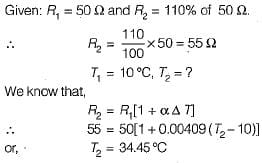QUESTION: 2

### A single-phase, two wire transmission line, 15 km long, is made up of round conductors, each 0.8 cm radius, separated from each other by 40 cm. The equivalent diameter of a fictitious hollow, thin-walled conductor having the same inductance as the original line is given by

Solution:

The fictitious conductor is one whose radius is r' and whose diameter is therefore,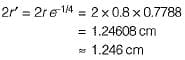QUESTION: 3

### If the conductor radius increases, inductance of the line,

Solution:

Inductance,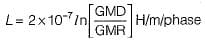Hence, if radius increases, GMR also increases. As a result of which inductance will decrease.

QUESTION: 4

For an underground cable, the surge impedance is generally taken as

Solution:

For underground cable, Zs = 40 Ω
For overhead line, Zs = 400 Ω

QUESTION: 5

Consider the following phenomenon occuring in a power system:
1. Corona loss
2. Charging current
3. Ferranti effect
4. Dielectric loss
5. Skin effect
Which of the above are associated with transmission lines?

Solution:

Dielectric loss occurs in underground cables not in a transmission line.

QUESTION: 6

The corona loss or the critical disruptive voltage in a transmission line at a temperature (f) and pressure (b) can be foundout by Using “air density correction factor (δ)” given by

Solution:
QUESTION: 7

A three-phase, 50 Hz, 400 kV, transmission line is 300 km long. The line inductance is 0.97 mH/km per phase and capacitance is 0.0115 μF/km per phase. Assuming a lossless line, the surge impedance of the line will be

Solution:

Surge impedance of the line is given by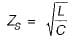or,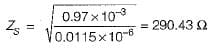QUESTION: 8

The resistance and reactance of a short line are equal. At zero regulation the load will be

Solution:

Zero regulation of a transmission line occurs at a leading power factor when φ = θ.
Since R = X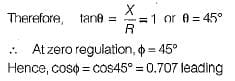QUESTION: 9

Shunt reactors are needed

Solution:

Shunt reactors are used to bring down receiving end voltage during light load or no-load condition to overcome Ferranti effect.

QUESTION: 10

Operating transmission lines close to their maximum power transfer capability will impair

Solution:Use Code STAYHOME200 and get INR 200 additional OFF Use Coupon Code